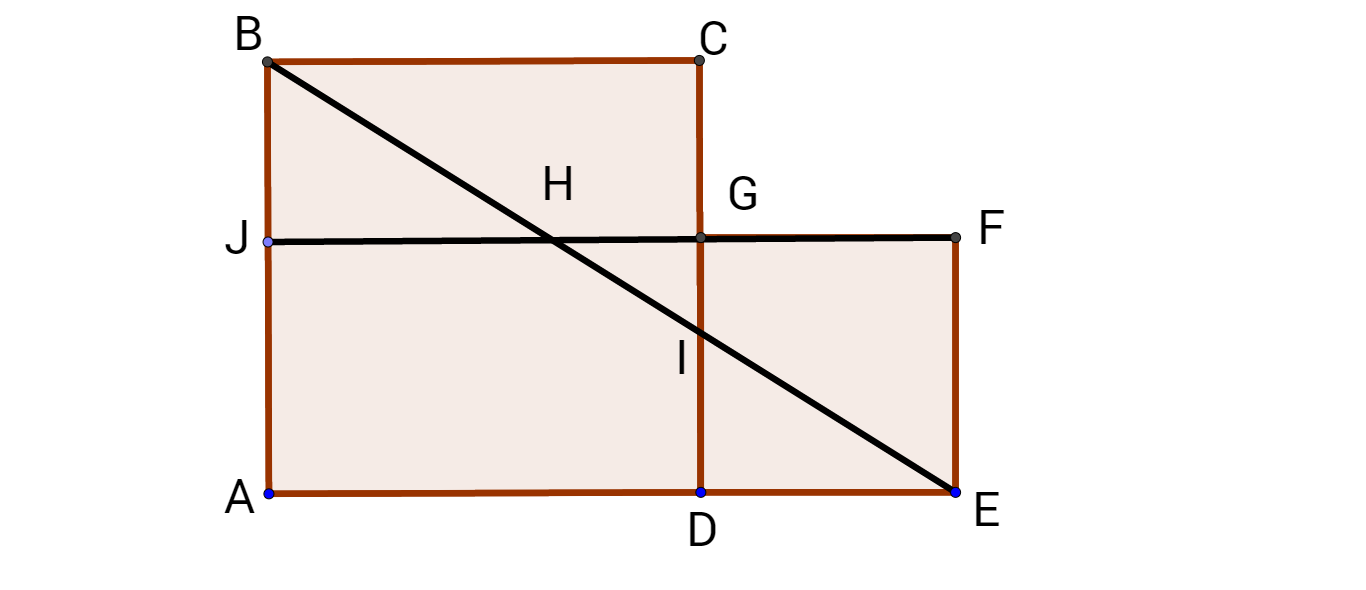# A geometry problem

Geometry Level 3In the figure above, $ABCD$ is a square and $AEFJ$ is a rectangle. If $JB=ID$ and $AB=111$. Find the area of the rectangle $AEFJ$.

×